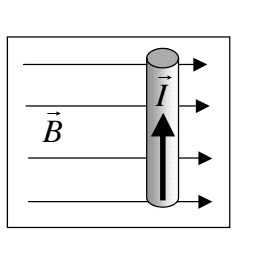# Using the right hand rule in physics1. Homework Statement

I need to use the right hand rule to determine the direction of the force on the current carrying wire shown in the above figure

## The Attempt at a Solution

Using the right hand rule, if I point my fingers in the same direction as I, then curl them towards B, would the force then be the direction my thumb is pointing, so into the page?

Looking for some help,

Thanks!

berkeman
Mentor
View attachment 207448
1. Homework Statement

I need to use the right hand rule to determine the direction of the force on the current carrying wire shown in the above figure

## The Attempt at a Solution

Using the right hand rule, if I point my fingers in the same direction as I, then curl them towards B, would the force then be the direction my thumb is pointing, so into the page?

Looking for some help,

Thanks!
Correct. F = qv X B

•J6204
Correct. F = qv X B
awesome thanks for the clarification!